# Signals and Systems: Even and Odd Signals

## Even Signal

A signal which is symmetrical about the vertical axis or time origin is known as even signal or even function. Therefore, the even signals are also called the symmetrical signals. Cosine wave is an example of even signal.

## Continuous-time Even Signal

A continuous-time signal x(t) is called the even signal or symmetrical signal if it satisfies the following condition,

𝑥(𝑡) = 𝑥(−𝑡); for − ∞ < 𝑡 < ∞

Some examples of continuous-time even signals are shown in Figure-1.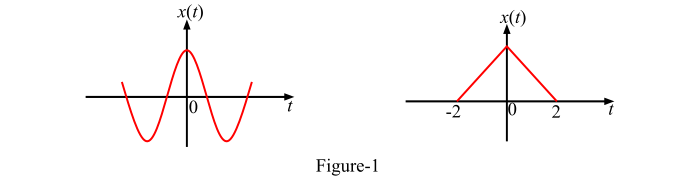## Discrete-time Even Signal

A discrete-time signal x(n) is said to be even signal or symmetrical signal if it satisfies the condition,

𝑥(𝑛) = 𝑥(−𝑛); for − ∞ < 𝑛 < ∞

Examples of discrete-time even signals are shown in Figure-2.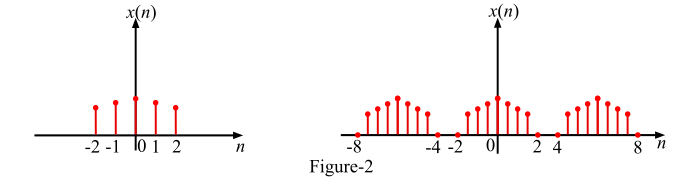## Properties of Even Signals

The properties of the even signals are given as follows −

• The even signals are symmetrical about the vertical axis.
• The value of an even signal at time (t) is same as at time (-t).
• The even signal is identical with its reflection about the origin.
• Area under the even signal is two time of its one side area.

## Odd Signal

A signal that is anti-symmetrical about the vertical axis is known as odd signal or odd function. Therefore, the odd signals are also called the antisymmetric signals. Sine wave is an example of odd signal.

## Continuous-time Odd Signal

A continuous time signal x(t) is called an odd signal or antisymmetric signal if it satisfies the following condition,

𝑥(−𝑡) = −𝑥(𝑡); for − ∞ < 𝑡 < ∞

Examples of continuous time odd signals or antisymmetric signals are shown in Figure-3.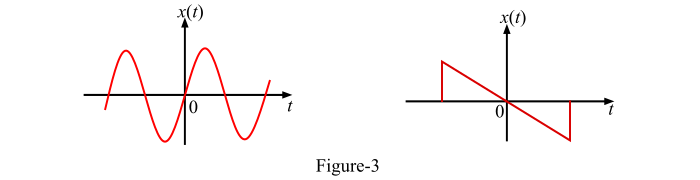## Discrete-time Odd Signal

A discrete time signal x(n) is said to be an odd signal or antisymmetric signal, if it satisfies the following condition,

𝑥(−𝑛) = −𝑥(𝑛); for − ∞ < 𝑛 < ∞

Examples of discrete-time odd signals are shown in Figure-4.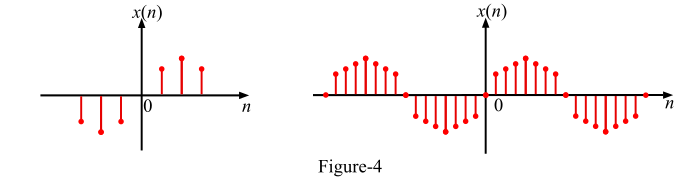## Properties of Odd Signals

Following are the properties of the odd signals −

• The odd signal is antisymmetric about the origin.

• The value of odd signal at time (t) is negative of its value at time (-t) for all t, i.e., −∞ < 𝑡 < ∞.

• The odd signal must necessarily be zero at time t = 0 to hold 𝑥(0) = −𝑥(0).

• Area under the odd signal is always zero.

Note – A continuous-time signal is said to be neither even nor odd if it does not satisfy the condition of the even signal and that of the odd signal. Some examples of such signals (neither even nor odd) are shown in Figure-5.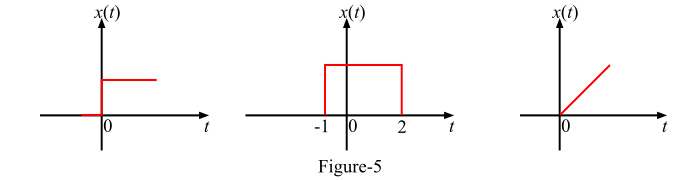## Numerical Example

Find whether the signals are even or odd.

• 𝑥(𝑡) = 𝑒−5𝑡

• 𝑥(𝑡) = sin 2𝑡

• 𝑥(𝑡) = cos 5𝑡

### Solution

• Given signal is,

𝑥(𝑡) = 𝑒−5𝑡

𝑥(−𝑡) = 𝑒5𝑡

−𝑥(𝑡) = −𝑒−5𝑡

It is clear that 𝑥(𝑡) ≠ 𝑥(−𝑡) and 𝑥(−𝑡) ≠ −𝑥(𝑡), thus the given signal is neither even signal nor odd signal.

• The given signal is,

𝑥(𝑡) = sin 2𝑡

𝑥(−𝑡) = −sin 2𝑡

−𝑥(𝑡) = −sin 2𝑡

Hence, 𝑥(𝑡) ≠ 𝑥(−𝑡); but 𝑥(−𝑡) = −𝑥(𝑡), thus the given signal is an odd signal.

• Given signal is,

𝑥(𝑡) = cos 5𝑡

𝑥(−𝑡) = cos 5𝑡

−𝑥(𝑡) = −cos 5𝑡

Therefore, 𝑥(𝑡) = 𝑥(−𝑡) and 𝑥(−𝑡) ≠ −𝑥(𝑡), thus the given signal is an even signal.